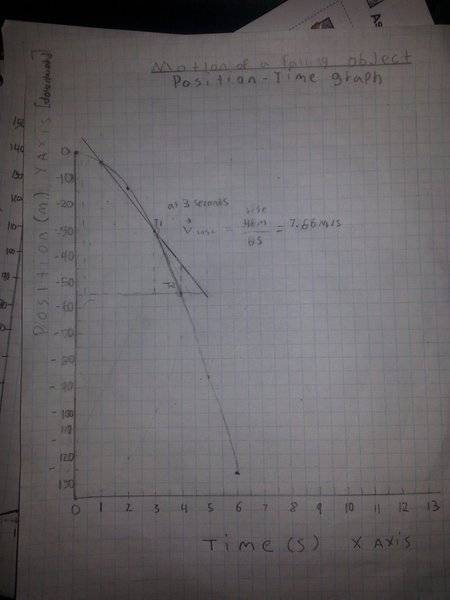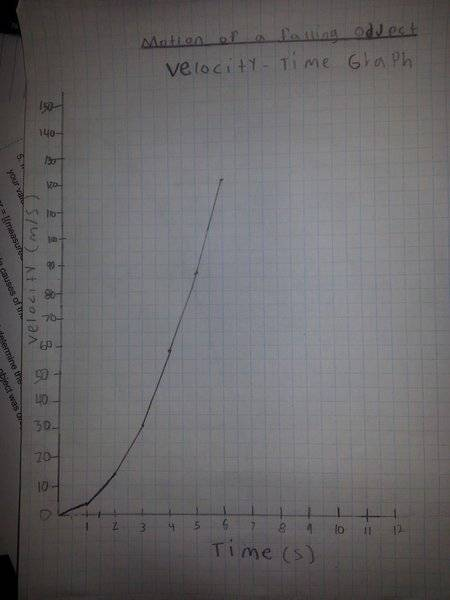# Position-time graph/velocity graph help

• Marleycake

## Homework Statement

Hi, I just started my kinetics course a few days ago and have a few questions about how to graph this and maybe help me out with some of the equations involved, I'm completely new to this and I got this test sheet a few days ago. thanks a lot guys
http://orig11.deviantart.net/afb9/f/2016/080/f/b/help_me_solve_these_people_and_i_ll_give_you_donat_by_marleycake-d9vydtd.png [Broken]

How do I graph this for the Position-Time Graph and my Velocity-Time Graph

for Position-Time Graph Do I start from the top of the graph (because it represents a free falling object) and make my curve or do I start from the bottom upward ?

## Homework Equations

my sheet also says

2. According to your position-time graph what type of motion did the object undergo?. this would be Instantaneous Velocity correct? or maybe I should put Constant Acceleration?

4. construct a V-T graph using your data. Draw the line of best fit. calculate the slope of the line. what doe the slope represent? Wouldn't their be more than one line, also the slope represents avrg velocity correct?
5. If accelerating is supposed to be 9.8 m/s2 [down], determine the percentage error of your value.
% of error ={(measured value ? accepted value)/accepted value] x 100.
could this be due to air resistance acting upon the falling object? also how would i go about calculating air resistance into my equation

6. State possible errors caused in your measured values. what techniques could be used to correct it? maybe incorrect calculations? so a calculator ?
7. Use your V-T graph, and determine the maximum displacement of the object, does this match the height from which the object falls.
I would say yes because if I'm correct my textbook says displacement is a vector and position is a scaler, the only direction the object goes is downward, so they would match? but how would I show that in my work

Last edited by a moderator:

Hello Marleycake,Here at PF we encourage initiative. Such as googling e.g. "position-time graph of a free falling object". Top of the list helps you on your way ! Further help is on the way when you post your first graph.

googling "position-time graph" gets you another useful link in the same site.

PS I like the exercise composers sense of humour. But what a waste of a nice bugatti ! Did they crash the Corvette too, or whas that moving horizontally ?

Ok so here are my graphs, is this correct way to graph them based on the picture, am I calculating the instantaneous velocity correcting, is my tangent line not correctly placed, also I've been searching on YouTube (& Google has helped but it kept showing examples of non free fall graphs on how to calculate the instantaneous velocity so I was a bit confused and off putting but I hope I did this correctly also still confused regarding the other questions but will proceed to Google them the best descriptive way I know howhere is the Velocity-Time GraphAlso thank you BvU for your encouragement

just realized my velocity time graph had to start downward too :( so i'll fix that ..another question is on the y axes should i put m/s/s considering its at a downward freefall? sorry for the billions of questions